# Lubricated friction:

## The 1D model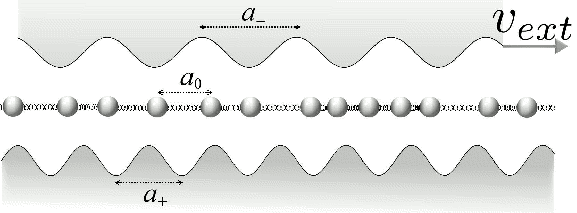We find a peculiar velocity quantization phenomenon in the classical motion of an idealized solid lubricant, consisting of a harmonic chain interposed between two incommensurate sliders, as sketched at the right. The ratio vcm/vext of the mean chain center-of-mass velocity to the externally imposed relative velocity of the sliders remains pinned to exact ``plateau'' values for wide ranges of parameters, such as sliders corrugation amplitudes, external velocity, chain stiffness and dissipation, and is strictly determined by one length ratio only.

The relevant lengths are:

a0 = lubricant rest periodicity

a+ = static substrate periodicity (taken as unit length)

a- = periodicity of substrate sliding at vext (=0.1 in all reported simulations)

These lengths define two lengthscale ratios r+=a+/a0 and r-=a-/a0. We assume, without loss of generality, r+ < r- and 1/r+ < r-.The plateau dynamics of the model is illustrated in the animation at the right, where the (+) potential is drawn in red, and the (-) potential is drawn in blue. The solid lubricant chain trapped between two sliding periodic substrates is represented by circles. The two sliders act as shifting cosine potentials on every particle in the chain. The resulting model is a generalization of the classical Frenkel-Kontorova model. The animation has r+=φ and r-=r+/(r+-1)=φ², with φ = the golden mean (GM) = (1+5½)/2≈1.61.

vcm/vext remains constant ("quantized") for variations of most model parameter, e.g. the restoring constant K of the springs joining the lubricant particles (of unit mass). K=2 in the animation.

In the model we include a phenomenologic dissipation term, with a viscous force on the jth particle equaling -γ(2 vj-vext). We take a small damping γ=0.1, to keep the dynamics underdamped. Note that this term favors sliding at speed vext/2, an intermediate and unbiased choice between sticking to the bottom (+) and the top (-) reference frame.

Any of several frames of reference can be chosen according to convenience to examine this dynamics. The figure example adopts the frame of reference where the potential (+) is static (v+=0 v-=vext). Here the plateau velocity is

vcm/vext = 1 - 1/r+.

### Understanding the plateau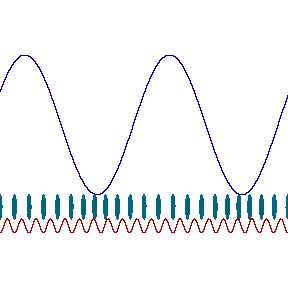The velocity plateaus are understood in terms of (soliton) density waves produced by density mismatch to the (+) potential and driven by the (-) potential. This point is clearest when r+ is near unity, as in the animation at the right, with r+=σ, with σ = 1+(π/310)½≈1.10. Once every 1/(r+-1) minima, an extra lubricant particle must "squeeze in" to preserve the extra mean density given by the slightly smaller a0 < a+. This extra density lump is precisely a soliton or kink: in this example a soliton occurs every about 10 lubricant particles.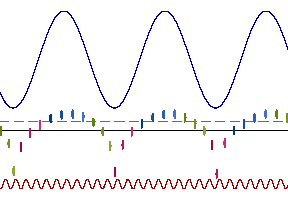One could visualize solitons more clearly by plotting again the particle position xi on the horizontal scale, and moving the particles vertically proportionally to the distance xi - xi-1 between neighboring particles (which is also the inverse of the local particle density). In this scheme, and in the (-) frame of reference, the previous animation becomes the animation at the right. One sees that neighboring particles move consistently closer in the correspondence of the minima of the upper (-) potential. These closeups are precisely the solitons (or kinks). Solitons pin to the (-) substrate, at its sliding velocity vext: in this pinned state the (-) frame of reference is the best one to visualize solitons.

In this animation, the dashed line represents the distance a+ at which the lubricant particles match the minima of the (+) substrate. The solid line represents the equilibrium interparticle separation a0, i.e. the inverse mean density of the lubricant.

As the lubricant becomes stiffer (larger spring K), solitons gradually expand in size (involving more particles), interact more and more strongly, and decrease in amplitude. Please see the animation for K=100 to clarify this point. Eventually, when the chain becomes too stiff, the solitons density amplitude is so small that it ceases to pin to the (-) substrate: the plateau is then lost, and the particle speed quits the perfect plateau value vcm/vext = 1 - 1/r+ (in the + reference). The animation of the non-plateau state K=300 shows an alternating formation and flattening out of the kinks lattice as it forces its way through the (-) corrugation.

On the opposite, soft-springs side, kinks melt away due to retardation: particles are just too slow to react and arrange their relative distance as required by the movement of the potentials, thus kinks get swept out, and the plateau ends. Of course, if vext is lowered from the 0.1 used in all calculations to, e.g. 0.01, then the plateau extends to softer chains. Additionally, the kinks formation mechanism (particles tend to stick to the minima of the (+) potential) is also altered if the amplitude of the (-) potential is larger than that of the (+) potential, and this can induce irregular motion and particles clustering, as illustrated in the animation of the non-plateau state K=0.5.

### Anti-solitons: the lubricant moves backward!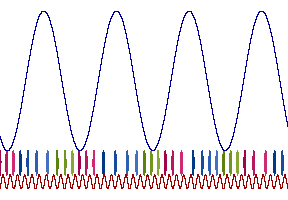More interestingly, when the particle density 1/a0=r+/a+ is lower than the density 1/a+ of minima of the (+) potential, r+<1, we realize a negative density (r+-1)/a+ of kinks. This can be seen as a positive density (1-r+)/a+ of antisolitons, or antikinks. Like kinks, antikinks pin to the (-) substrate, with the effect of also producing a particles velocity vcm/vext = 1 - 1/r+<0 (in the (+) frame)! This is illustrated by the figure at the right (where r+=89/100, r-=101½·100/89, and the (+)-potential frame is selected): exactly like holes in a semiconductor, anti-kinks (carrying a negative ``charge'') moving at velocity +vext effectively produce a net backward lubricant motion: particles on average do move to the left, even though the dragging substrate (-) moves to the right.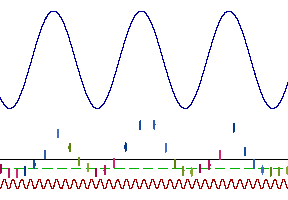Like for kinks, the anti-kink quantized motion is best seen in the (-) frame of reference, again putting the inter-particle separation along the vertical direction. The animation at the right illustrates the anti-kink movement: "peaks" of increased interparticle separation (less dense lubricant) remain pinned to the maxima of the (-) potential.

In all previous examples we selected r-=r+/(r+-1) as a convenient choice, since the distance between solitons matches the distance between minima of the (-) potential. In the present example instead the choice of r- is generic, which means that the antikink densities and (-)-potential minima density are incommensurate, so that the anti-kinks arrange irregularly and do not form a perfect lattice. With this generic r-, the density (1-r+)/a+=0.11/a+ of antikinks exceeds the density 1/a- = r+/(r-a+)=0.0995../a+ of (-) minima. Accordingly, occasionally a pair of antikinks pins to a single (-) maximum, as e.g. near the center of the figure at the right.

### The plateau limits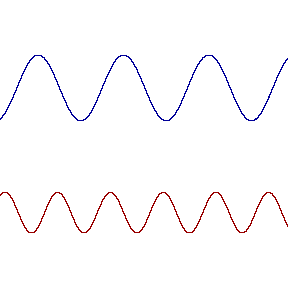Simulations show that this soliton physics is so robust that it survives even to regimes r+ > 2 and r+ < 0.5 where one could not tell individual solitons apart. Also, finite size effect do not destroy this solitonic dynamics, as the finite (and very small!) size of the chain at the right demonstrates.

All nice things eventually come to an end, of course! The plateau state is lost as K becomes either too small or too large, as discussed above. The plateau is also destroyed by a very large sliding velocity vext, which does not leave the kinks time to form and pin to the (-) substrate.

Also the application of an external force (acting on all particles) can lead to an unpinned state. If this force Fext is large enough it makes the particles slide quickly, thus melting the kinks lattice, or at least making it slip against the (-) substrate. We observe a hysteretical behavior when we ramp up as the force slowly to the unpinned state and back down into the dynamical plateau state. The model shows an intermediate-force region of coexistence of pinned and unpinned behavior for the same Fext, depending on the initial state. For example, see the animation of the pinned state obtained increasing Fext to 0.012 and the animation of unpinned state obtained with the same Fext=0.012, but obtained by decreasing Fext from the large-Fext unpinned state. Upon further decrease of the force to Fext=0.0068828, the solitons remain unpinned, but intermittencies occur less frequently, and the speed approaches slowly the plateau one. These 3 calculations have K=100 r+=σ, r-=r+/(r+-1) as above. For more details about this hysteresis, see [cond-mat/0609117]

### The sound of friction

The oscillatory motions of the particles about their average drift can even be heard as audio files. The smoother, more harmonic oscillation for K=10 results in a smoother sound than the sharper bouncier K=1 motion, which is richer in harmonic contents. As the auditive sensation, and a more detailed Fourier analysis demonstrates, the oscillations of the particles around their average drift is periodic!

Nonperiodic (or rather quasi periodic) oscillations of the individual lubricant particles are found for other incommensuracy ratios. Hear for example the nonperiodic sounds of π/2.

## Extension beyond 1D

We find evidence of the quantized plateau dynamics also in a 2D model where the lubricant atoms can move also transversally to the sliding direction. The plateau velocity is supported by a lubricant as many as 6-layers thick.

We also find evidence of the quantized lubricant motion in a fully 3D model, based on Lennard-Jones interactions. We even detect the quantized-sliding phenomenon for a graphene lubricant layer, as reported in this paper. Incommensuracy could even arise in lattice-matched conditions, by means of mutual rotation, as in this movie.

## Implications for experiments

The impressive robustness of the velocity plateaus, due to their topological nature should be realized in nature, but need not be especially easy to observe, especially since it is a property of the lubricant dynamics, which is usually hard to probe. However, the plateau dynamics has tribological implications: frictional forces, those opposing the advancement of the (-) substrate decrease sharply as the quantized-sliding regime starts.

On the other hand, irregular asymmetric sliding of the lubricant can have potentially disruptive effects on lubricated friction in a macroscopical multi-grain context.

To date, we are aware of no clear experimental evidence of the velocity-plateau phenomenon.

A number of local theses and international publications report different sides of this kink-dragging mechanism, including:created: 7 Mar 2006